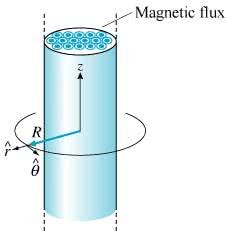1
0
watching
1,169
views10 Nov 2019

#Learning Goal: To work through astraightforward application of Faraday's law to find the EMF andthe electric field surrounding a region of increasing fluxFaraday's law describes how electric fields and electromotiveforces are generated from changing magnetic fields. This problem isa prototypical example in which an increasing magnetic fluxgenerates a finite line integral of the electric field around aclosed loop that surrounds the changing magnetic flux through asurface bounded by that loop. A cylindrical iron rod withcross-sectional area is oriented with its symmetry axis coincidentwith the z axis of a cylindrical coordinate system as shown. It hasa uniform magnetic field inside that varies according to . In otherwords, the magentic field is always in the positive z direction,and it has no other components.For your convenience, we restate Faraday's law here: , where is theline integral of the electric field, and the magnetic flux is givenby , where is the angle between the magnetic field and the localnormal to the surface bounded by the closed loop.Direction: The line integral and surface integral reverse theirsigns if the reference direction of or is reversed. The right-handrule applies here: If the thumb of your right hand is taken along ,then the fingers point along . You are free to take the loopanywhere you choose, although usually it makes sense to choose itto lie along the path of the circuit you are considering.Find , the electromotive force (EMF) around a loop that is atdistance from the z axis, where is restricted to the region outsidethe iron rod as shown. Take the direction shown in the figure aspositive.Express in terms of , , , , and any needed constants such as , ,and .Due to the cylindrical symmetry of this problem, the inducedelectric field can depend only on the distance from the z axis,where is restricted to the region outside the iron rod. Find thisfield.Express in terms of quantities given in the introduction (andconstants), using the unit vectors in the cylindrical coordinatesystem,r^ ,theta^ , and z^ .